# Arccosine: Definition & Overview

Lesson Transcript
Instructor: Jennifer Beddoe

Jennifer has an MS in Chemistry and a BS in Biological Sciences.

Arccosine is an inverse trigonometric function. It happens to be the inverse of cosine and can be used to solve more complicated right angle problems. This lesson will define the arccosine in more detail and give some example problems. Be prepared for a quiz at the end of the lesson. Updated: 08/31/2021

## Inverse Operations

In mathematics, most operations have an inverse operation, which is the opposite of the original operation. For example, subtraction is the inverse of addition, and multiplication is the opposite of division.

Trigonometric functions are no different. They all have inverse operations. Here is a table of the functions and inverse functions:

Function Inverse Function
sine arcsine
cosine arccosine
tangent arctangent
secant arcsecant
cosecant arccosecant
cotangent arccotangent

Inverse trigonometric functions have practical applications in physics, engineering, and navigation.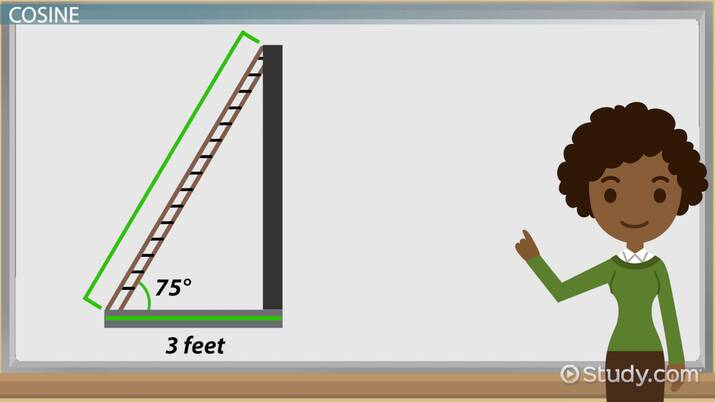An error occurred trying to load this video.

Try refreshing the page, or contact customer support.

Coming up next: Arcsine: Definition & Overview

### You're on a roll. Keep up the good work!

Replay
Your next lesson will play in 10 seconds
• 0:04 Inverse Operations
• 0:33 Cosine
• 1:33 Arccosine
• 2:03 Examples
• 3:14 Lesson Summary
Save Save

Want to watch this again later?

Timeline
Autoplay
Autoplay
Speed Speed

## Cosine

The cosine (cos) of an angle in a right triangle is a trigonometric function that can be defined as the ratio of the side adjacent to the angle and the hypotenuse.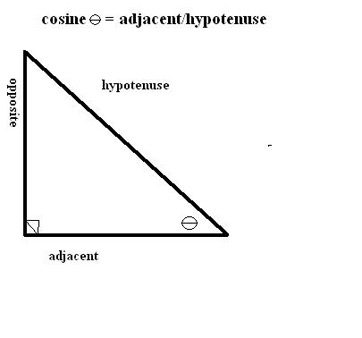The graph of the cosine function looks like this: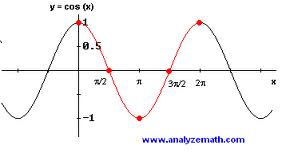The cosine function can be used to solve problems involving a right triangle. For example: Say there is a ladder leaning against the side of a building. You want to know how tall the ladder is without moving it or climbing it. You know that the base of the ladder is 3 feet from the building and that the angle the ladder makes with the ground is 75°. You can use the cosine function to determine the length of the ladder.

cos(75) = 3ft / hypotenuse

hypotenuse = 3 ft / cos75

hypotenuse = 3 / 0.25882

hypotenuse = 11.59 ft

So the ladder is 11.59 ft tall.

## Arccosine

The arccosine is the inverse of the cosine. It's most useful when trying to find the angle measure when two sides of a triangle are known.

The graph of the arccosine looks like this: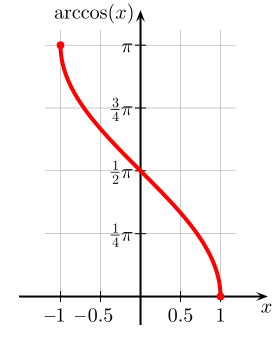To solve problems using the arccosine, you need a scientific calculator. It should have a button, usually above the cosine button that looks like this: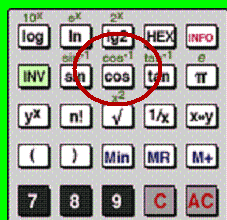The arccosine allows you to find the measure of an angle when you know the ratio of the adjacent side to the hypotenuse.

To unlock this lesson you must be a Study.com Member.

### Register to view this lesson

Are you a student or a teacher?